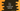# How to find compound interest in C++## How to find compound interest in C++:

In this post, we will learn how to find the compound interest in C++. Compound interest is also called interest on interest. It is calculated based on the initial principal value and the accumulated interests from past periods. It makes the sum amount grow at a faster rate than simple interest.

Compound interest calculation is easy if you know the formula. In this post, we will learn the formula to calculate compound interest and how to do that with examples.

## Formula to calculate compound interest:

Compound interest can be calculated by using the following formula:

``A = P * (1 + r/n)^(nt)``

Here, A - It is the final amount, it includes both compound interest and the initial amount. P - It is the principal amount, or the initial amount. r - It is interest rate. n - It is the number of times interest is applied for each time period. t - It is the number of time periods.

By using this formula, we will get the final amount. But it includes both compound interest and principal amount. So, if we need to calculate only the interest, we can do this by subtracting P from this value.

``Interest = P * (1 + r/n)^(nt) - P``

## C++ program to calculate compound interest:

Let’s write the above formula in code:

``````#include <iostream>
#include <cmath>
using namespace std;

int main()
{
float A, P, r, n = 1, t;
cout << "Enter the principal amount: " << endl;
cin >> P;

cout << "Enter the rate of interest: " << endl;
cin >> r;

r = r / 100;

cout << "Enter the time period: " << endl;
cin >> t;

A = P * pow((1 + r / n), n * t);

cout << "Final Amount: " << A << endl;
cout << "Compound interest: " << A - P << endl;

return 0;
}``````

### Explanation:

In this program,

• We have initialized five variables A, P, r, n, t to store the final amount, principal amount, rate of interest, number of times interest is calculated per period and time period. The value of n is assigned as 1, i.e. interest is calculated once per period.
• It asks the user to enter the values and stores them in the variables. r is changed to r/100 because it is the rate of interest. If user enteres 12, it will be 0.12 that we need to use in the formula.
• The final amount is calculated by using the formula we discussed above. It is stored in the variable A. For calculating the power value, pow method is used which is defined in cmath header file.
• Compound interest is also calculated by subtracting P from A.

### Sample output:

If you run this program, it will print output as like below:

``````Enter the principal amount:
100
Enter the rate of interest:
10
Enter the time period:
10
Final Amount: 259.374
Compound interest: 159.374

Enter the principal amount:
1000
Enter the rate of interest:
24
Enter the time period:
20
Final Amount: 73864.2
Compound interest: 72864.2

Enter the principal amount:
2399
Enter the rate of interest:
23.5
Enter the time period:
20
Final Amount: 163444
Compound interest: 161045``````